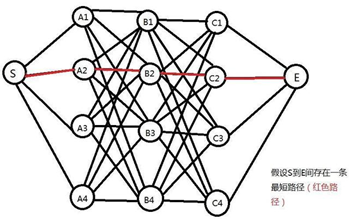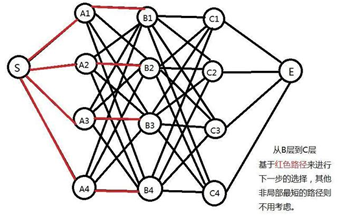维特比算法（Viterbi）及python实现样例step1：从点S出发。对于第一层的4个节点，算出他们的距离d(S,A1),d(S,A2),d(S,A3),d(S,A4),因为只有一步，所以这些距离都是S到它们各自的最短距离

step2:对于B层的所有节点(B1,B2,B3,B4),要计算出S到他们的最短距离。我们知道，对于特定的节点B2，从S到它的路径可以经过A层的任何一个节点(A1,A2,A3,A4)。对应的路径长就是d(S,B2)=d(S,Ai)+d(Ai,B2)（其中i=1，2，3，4）。由于A层有4个节点（即i有4个取值），我们要一一计算，然后找到最小值。这样，对于B层的每个节点，都需要进行4次运算，而B层有4个节点，所以共有4*4=16次运算。

step3:这一步是该算法的核心。我们从step2计算得出的阶段结果只保留4个最短路径值（每个节点保留一个）。那么，若从B层走向C层来说，该步骤的级数已经不再是4*4，而是变成4！也就是说，从B层到C层的最短路径只需要基于B层得出的4个结果来计算。这种方法一直持续到最后一个状态，每一步计算的复杂度为相邻两层的计算复杂度为4*4乘积的正比！再通俗点说，连接着两两相邻层的计算符合变成了“+”号，取代了原先的“*”号。用这种方法，只需要进行4*4*2=32次计算！

1、  题目背景

2、  已知情况

}

}

3、  已知上述，求：阿鲁这三天的身体健康状态变换的过程是怎么样的？

4、  过程：

1.初始情况：

P(健康) = 0.6，P(发烧)=0.4。

2.求第一天的身体情况：

P(今天健康) = P(正常|健康)*P(健康|初始情况) = 0.5 * 0.6 = 0.3

P(今天发烧) = P(正常|发烧)*P(发烧|初始情况) = 0.1 * 0.4 = 0.04

3.求第二天的身体状况：

P(前一天发烧，今天发烧) = P(前一天发烧)*P(发烧->发烧)*P(冷|发烧) = 0.04 * 0.6 * 0.3 = 0.0072

P(前一天发烧，今天健康) = P(前一天发烧)*P(发烧->健康)*P(冷|健康) = 0.04 * 0.4 * 0.4 = 0.0064

P(前一天健康，今天健康) = P(前一天健康)*P(健康->健康)*P(冷|健康) = 0.3 * 0.7 * 0.4 = 0.084

P(前一天健康，今天发烧) = P(前一天健康)*P(健康->发烧)*P(冷|发烧) = 0.3 * 0.3 *.03 = 0.027

4.求第三天的身体状态：

P(前一天发烧，今天发烧) = P(前一天发烧)*P(发烧->发烧)*P(头晕|发烧) = 0.027 * 0.6 * 0.6 = 0.00972

P(前一天发烧，今天健康) = P(前一天发烧)*P(发烧->健康)*P(头晕|健康) = 0.027 * 0.4 * 0.1 = 0.00108

P(前一天健康，今天健康) = P(前一天健康)*P(健康->健康)*P(头晕|健康) = 0.084 * 0.7 * 0.1 = 0.00588

P(前一天健康，今天发烧) = P(前一天健康)*P(健康->发烧)*P(头晕|发烧) = 0.084 * 0.3 *0.6 = 0.01512

5.结论

1 import numpy as np
2 def viterbi(trainsition_probability,emission_probability,pi,obs_seq):
3     #转换为矩阵进行运算
4     trainsition_probability=np.array(trainsition_probability)
5     emission_probability=np.array(emission_probability)
6     pi=np.array(pi)
7     obs_seq = [0, 2, 3]
8     # 最后返回一个Row*Col的矩阵结果
9     Row = np.array(trainsition_probability).shape
10     Col = len(obs_seq)
11     #定义要返回的矩阵
12     F=np.zeros((Row,Col))
13     #初始状态
14     F[:,0]=pi*np.transpose(emission_probability[:,obs_seq])
15     for t in range(1,Col):
16         list_max=[]
17         for n in range(Row):
18             list_x=list(np.array(F[:,t-1])*np.transpose(trainsition_probability[:,n]))
19             #获取最大概率
20             list_p=[]
21             for i in list_x:
22                 list_p.append(i*10000)
23             list_max.append(max(list_p)/10000)
24         F[:,t]=np.array(list_max)*np.transpose(emission_probability[:,obs_seq[t]])
25     return F
26
27 if __name__=='__main__':
28     #隐藏状态
29     invisible=['Sunny','Cloud','Rainy']
30     #初始状态
31     pi=[0.63,0.17,0.20]
32     #转移矩阵
33     trainsion_probility=[[0.5,0.375,0.125],[0.25,0.125,0.625],[0.25,0.375,0.375]]
34     #发射矩阵
35     emission_probility=[[0.6,0.2,0.15,0.05],[0.25,0.25,0.25,0.25],[0.05,0.10,0.35,0.5]]
36     #最后显示状态
37     obs_seq=[0,2,3]
38     #最后返回一个Row*Col的矩阵结果
39     F=viterbi(trainsion_probility,emission_probility,pi,obs_seq)
40     print(F)

[[ 0.378　　   0.02835　　      0.00070875]
[ 0.0425 　　 0.0354375 　　 0.00265781]
[ 0.01 　　     0.0165375 　　 0.01107422]]

posted @ 2018-07-30 15:54  我们都是大好青年  阅读(18386)  评论(4编辑  收藏  举报